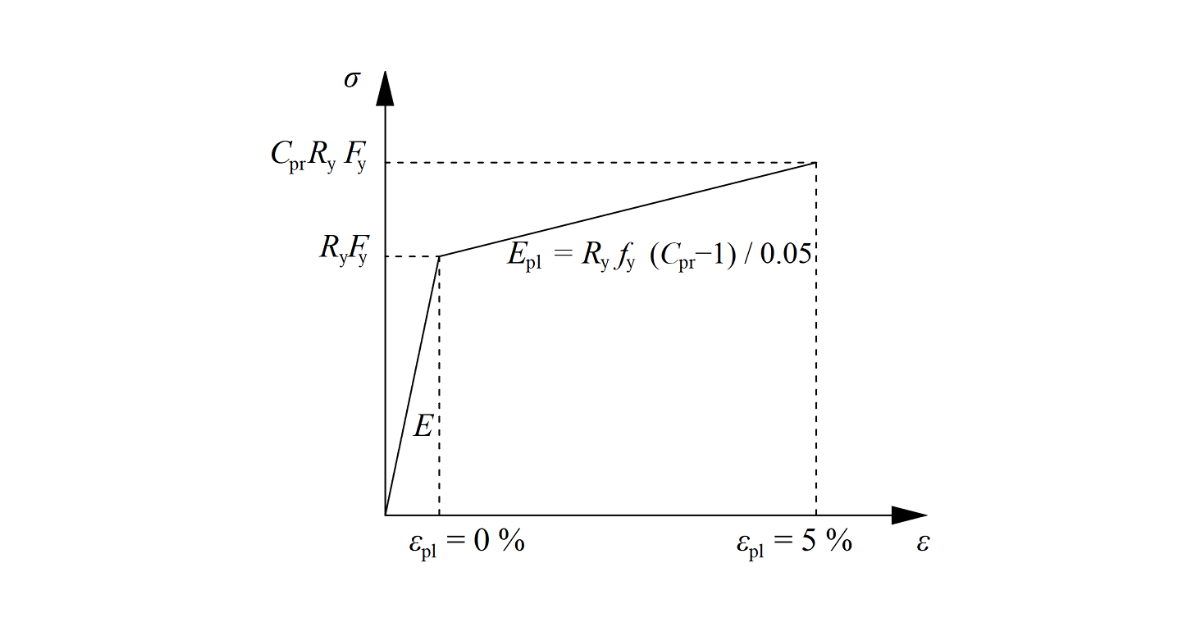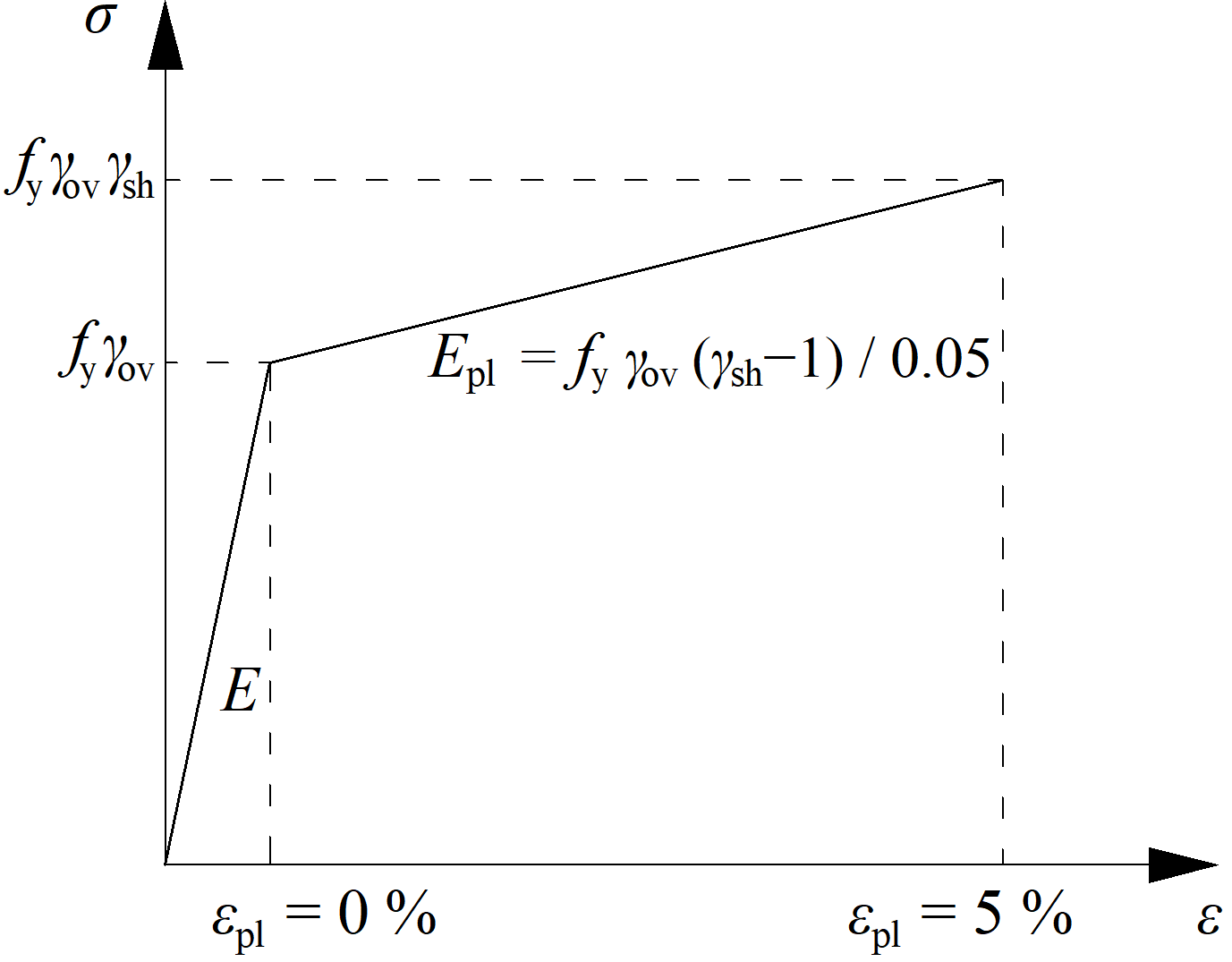### Choose language# Capacity design according to Indian Standard

The objective of capacity design is to confirm a building undergoes controlled ductile behaviour in order to avoid collapse in a design-level earthquake.
$$Plastic hinge is expected to appear in dissipative item and all non-dissipative items of the joint must be able to safely transfer forces due to the yielding in the dissipative item. The dissipative item is usually a beam in moment resisting frame. The safety factor is not used for dissipative items:Two factors are assigned to the dissipative item: $$\gamma_{ov}$$ – overstrength factor – IS 800, Cl. 12; the recommended value is $$\gamma_{ov} = 1.2$$; editable in materials $$\gamma_{sh}$$ – strain-hardening factor; the recommended value is $$\gamma_{sh} = 1.0$$; editable in operationThe increased strength of the dissipative item allows for the input of loads that cause the plastic hinge to appear in the dissipative item. In the case of moment resisting frame and beam as the dissipative item, the beam should be loaded by $$M_{y,Ed} = \gamma_{ov} \gamma_{sh} f_y W_{pl,y}$$ and corresponding shear force $$V_{z,Ed} = -2 M_{y,Ed} / L_h$$, where: $$f_y$$ – characteristic yield strength $$W_{pl,y}$$ – plastic section modulus $$L_h$$ – distance between plastic hinges on the beam In the case of an asymmetric joint, the beam should be loaded by both sagging and hogging bending moments and their corresponding shear forces.The plates of dissipative items are excluded from the check.$$